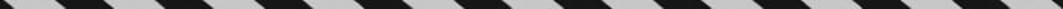﻿ ﻿PERCENT INCREASE AND DECREASE - Fractions, Decimals, and Percents - TOPICS IN ARITHMETIC - SAT SUBJECT TEST MATH LEVEL 1

## TOPICS IN ARITHMETIC## CHAPTER 3Fractions, Decimals, and Percents### PERCENT INCREASE AND DECREASE

Key Fact B12

The percent increase of a quantity isThe percent decrease of a quantity isEXAMPLE 13: From 1980 to 1990, the population of a town increased from 12,000 to 15,000. Since the actual increase in the population was 3,000, the percent increase in the population wasEXAMPLE 14: If from 1980 to 1990 the population of a town increased from 12,000 to 15,000 and from 1990 to 2000 the population increased by the same percent, then what was the percent increase in population from 1980 to 2000?

In Example 13, you calculated that from 1980 to 1990 the town”s population increased by 25%. If from 1990 to 2000 the population again increased by 25%, it increased by (0.25)(15,000) = 3,750. So, the population in 2000 was 18,750. Therefore, from 1980 to 2000, the population increased by 18,750 – 12,000 = 6,750. The percent increase wasNotice that a 25% increase followed by a second 25% increase is not a 50% increase.

Key Fact B13

An increase of a% followed by an increase of b% always results in a larger increase than a single increase of (a + b)%. Similarly, a decrease of a% followed by a decrease of b% always results in a smaller decrease than a single decrease of (a + b)%.

Key Fact B14

To increase a number by r %, multiply it by (1 + r %). To decrease a number by r %, multiply it by (1r %).

EXAMPLE 15: Starting on January 1, 1990, the population of Centerville increased by 5% every year. If Centerville”s population was 10,000 on January 1, 1990, what was its population on January 1, 1992?

Since the population on January 1, 1990 was 10,000, to find the population one year later on January 1, 1991, multiply by 1.05:

(1 + 5%)(10,000) = (1 + 0.05)(10,000) = (1.05)(10,000)=10,500To get the population on January 1, 1992, again multiply by 1.05.

(1.05)(10,500) = 11,025

Note that (1.05)(10,500) = (1.05)[(1.05)(10,000)] = (1.05)2(10,000). This process can be continued for any number of years.

Key Fact B15

If an initial quantity A increases r% per year, then the amount at the end of t years is given by A(t ) = A(1 + r %)t.

EXAMPLE 16: If the population of Centerville (which was 10,000 on January 1, 1990) grew at a rate of 5% per year for 10 years, what was its population on January 1, 2000?

The population after 10 years wasA(10) = 10,000(1 + 0.05)10 = 10,000(1.05)10 = 10,000(1.6289) = 16,289.

Note that 10 increases of 5% resulted not in a 50% increase, but in a total increase of 6,289 or nearly 63%. Also note that the population on January 1, 2000 was 163% of the population on January 1, 1990. Similarly, if the area of one square is 3 and the area of a second square is 18, the area of the larger square is 600% of the area of the smaller square:However, the area of the large square is 500% greater than the area of the small square. The increase in area from the small square to the large one is 15, and﻿